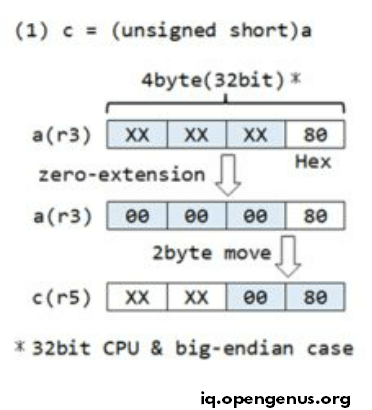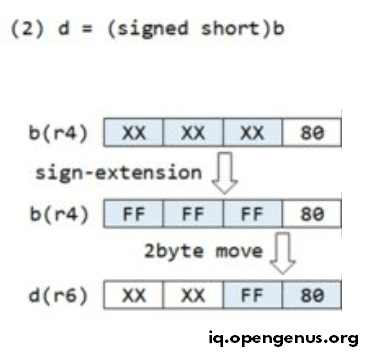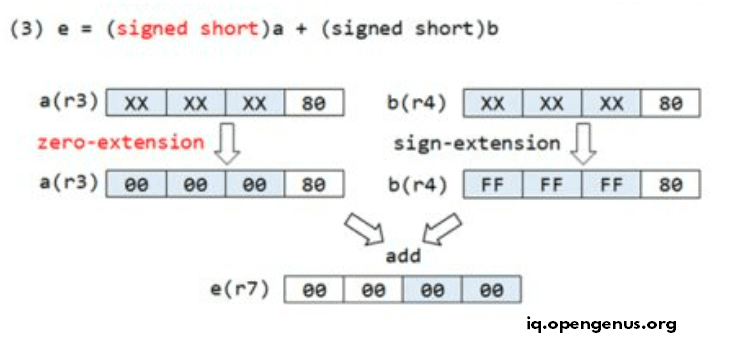×

Search anything:

# CPU operation of cast in C [zero and sign-extended]

#### C Programming Software EngineeringGet this book -> Problems on Array: For Interviews and Competitive Programming

In this article, we will understand how casting operates on the CPU level in C Programming Language along with the concept of zero-extension and sign-extension. This is a core concept in C Programming and Embedded Programming.

We will understand the concept with the help of an example:

Consider the following C code:

``````unsigned char a = 0x80;
signed short b = 0x80;
unsigned short c = 0x80;
signed short d = 0x80;
signed short e = 0x80;
``````

Local variables a, b, c, d, and e shall be set in general-purpose registers r3, r4, r5, r6, and r7.

We do the following 3 operations in C:

``````c = (unsigned short)a; // (1)
d = (signed short) b;   // (2)
e = (signed short)a + (signed short)b; // (3)
``````

## Line 1

``````c = (unsigned short)a; // (1)
``````

This image demonstrates the process:Observe the concept of zero-extension.

• The cast of a(r3) from unsigned char to unsigned short is zero-extended to match the CPU register length.
• Assign 2 Byte data to c(r5).

## Line 2

``````d = (signed short) b;   // (2)
``````

This image demonstrates the process:Observe the concept of sign-extension.

• The cast of b(r4) from signed char to signed short is sign-extended to match the CPU register length.
• Assign 2 Byte data to d(r6).

## Line 3

``````e = (signed short)a + (signed short)b; // (3)
``````

This image demonstrates the process:Revise the concept of zero-extension and sign-extension.

• The cast of a(r3) from unsigned char to signed short is zero-extended to match the CPU register length.
• The data extension method is determined by the declaration data type, not the cast specification type.
• The cast of b(r4) from signed char to signed short is sign-extended to match the CPU register length.
``````e(r7) = a(r3) + b(r4)
``````

With this article at OpenGenus, you must have the complete idea of CPU operation of cast in C along with the concept of zero-extended and sign-extended.#### Benjamin QoChuk, PhD

Benjamin QoChuk is a Computer Science Researcher, Inventor and Author. His educational background include Bachelors at Vanderbilt University and PhD in Computer Science at Peking University.